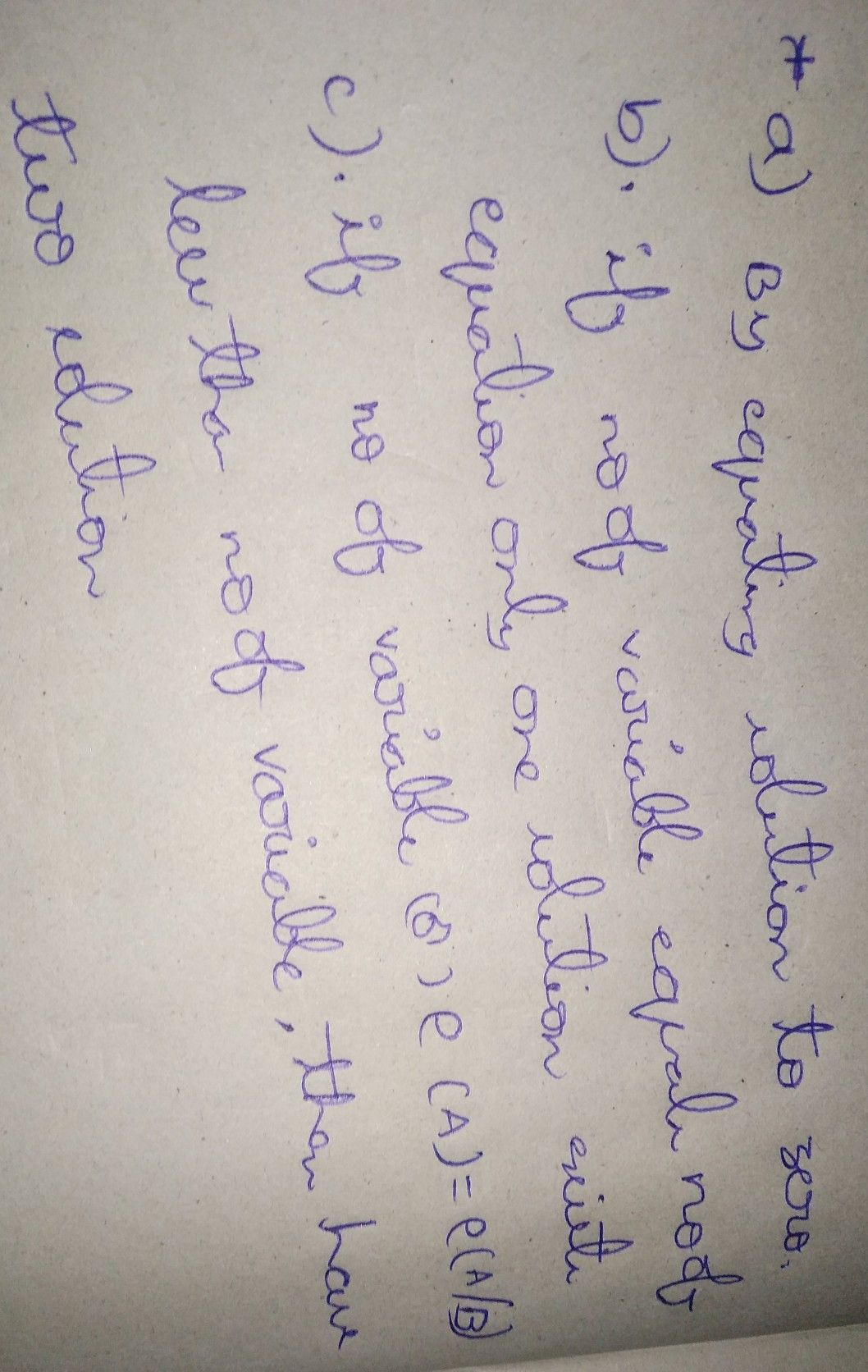Symbol
ProblemProcess $Ot/os//oms$ a. How did you find the solution(s) $\left(s\right)$ of each equation? b. Which of the equations has only one solution? Why? 6. Which of the equations has $//0$ solutions? Why? $23$
7th-9th grade
Other
Search count: 108
Question content
answer it in sentence form please
SolutionQanda teacher - Aravind